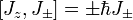Nezva
Calculate [Lz,L+]

L+=Lx+iLy
L-=Lx-iLy

Important Relations:
LxLy = i$$\hbar$$Lz, LyLz = i$$\hbar$$Lx, LzLx = i$$\hbar$$Ly

Lx = ypz - zpy, Ly = xpz - zpx, Lz = xpy - ypx

To start solving;
[Lz,L+]
Lz - (Lx + iLy) = 0
Multiply through by $$\hbar$$:
$$\hbar$$Lz - $$\hbar$$Lx + i$$\hbar$$Ly

The i$$\hbar$$Ly is equal to LzLx. From this point
I've tried varying approaches in attempt to cancel variable out, but have failed. I have a feeling this problem can be solved easier. Should I try to use spherical coordinates instead of Cartesian? From trying to figure this out I have stumbled upon the answer but I would like to know how to produce the answer.Last edited by a moderator:

To start solving;
[Lz,L+]
Lz - (Lx + iLy) = 0

This is not even close to correct ... where did you get that zero from? Where are the products of the bracketed operators?

The i$$\hbar$$Ly is equal to LzLx.

The above is not correct ... the correct relation is:

$$i\hbar L_{y}=[L_{z},L_{x}]$$

where the bracketed term on the right is the commutator of Lz and Lx. (the initial thing you are solving for is also a commutator).

Do you know how to expand a commutator? Do you understand it's significance in QM? That is the place to start on this problem.

Last edited:
Nezva
Didn't even recognize that, thanks.

Starting over using the correct commutator rules: AB - BA = 0
LzL+-L+Lz=0 replacing L+ with Lx+iLy and multiplying the Lz through

=> LzLx+iLzLy-LxLz-iLyLz

Could you show me where you found the relation you used. I found a very similar one with the hbar in it.

Didn't even recognize that, thanks.

Starting over using the correct commutator rules: AB - BA = 0
LzL+-L+Lz=0 replacing L+ with Lx+iLy and multiplying the Lz through

=> LzLx+iLzLy-LxLz-iLyLz

Could you show me where you found the relation you used. I found a very similar one with the hbar in it.

Yeah ... mine should have an hbar in it too ... sorry about that .. it is fixed now.

Gold Member
Didn't even recognize that, thanks.

Starting over using the correct commutator rules: AB - BA = 0
LzL+-L+Lz=0 replacing L+ with Lx+iLy and multiplying the Lz through

=> LzLx+iLzLy-LxLz-iLyLz

Could you show me where you found the relation you used. I found a very similar one with the hbar in it.

AB-BA does not equal 0 in general. It only equals zero if A and B commute. Otherwise AB-BA=[A,B] that you calculated earlier.

Nezva
Does LzLx = LxLz ? I want to know if the relations hold true for both of them or if I have to add a negative sign (or something of that nature). Don't angular momentums commute?

Nezva
AB-BA does not equal 0 in general. It only equals zero if A and B commute. Otherwise AB-BA=[A,B] that you calculated earlier.

Thanks for the clarification. It's a measurement of the extent to which they do NOT commute, correct? I do not see where a non-zero commutator would be useful though.

Does LzLx = LxLz ? I want to know if the relations hold true for both of them or if I have to add a negative sign (or something of that nature). Don't angular momentums commute?

No, the different components of angular momentum along the cartesian axes most certainly do not commute. You can verify this for your self using the definition of angular momentum in terms of the cross product of the position and momentum vectors. Just replace the classical momenta with the corresponding QM operators and you will see that in general:

$$[L_{a},L_{b}]=\pm i\hbar L_{c}$$

where a, b and c are all different Cartesian axes. The sign will change based on the ordering of the of the axes ... if you have them ordered as xyzxyzxyz... it will be positive, if you have them ordered as zyxzyxzyx... it will be negative. (This can be stated very simply using a symbol called the Levi-Civita symbol, but that is a side issue .. google it if you are interested)

Nezva said:
Thanks for the clarification. It's a measurement of the extent to which they do NOT commute, correct? I do not see where a non-zero commutator would be useful though.

Good gracious! Haven't you studied the Heisenberg uncertainty principle yet? Quite simply, much of the weirdness of QM can be traced back to the fact that the properties of quantum states must inevitably give rise to non-commuting operators. The standard HUP formulation in terms of position and momentum arises because those two operators don't commute.

Nezva
Just replace the classical momenta with the corresponding QM operators and you will see that in general

$$[L_{a},L_{b}]=\pm i\hbar L_{c}$$

So that's where that comes from ;) I'm having trouble identifying all the equations from very similar ones. You seem to have a keen grasp of QM, where did you learn from? Some kind of organization in this maelstrom of QM ideas and variables would be nice...

(This can be stated very simply using a symbol called the Levi-Civita symbol, but that is a side issue .. google it if you are interested)

I've seen similar ideas but never a name for it. Thanks for that.

Good gracious! Haven't you studied the Heisenberg uncertainty principle yet? Quite simply, much of the weirdness of QM can be traced back to the fact that the properties of quantum states must inevitably give rise to non-commuting operators. The standard HUP formulation in terms of position and momentum arises because those two operators don't commute.

Ah sorry, I didn't realize HUP was a commutation. I simply memorized the formula.